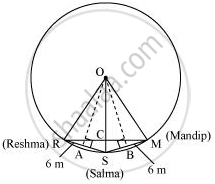# Three girls Reshma, Salma and Mandip are playing a game by standing on a circle of radius 5m drawn in a park. Reshma throws a ball to Salma, Salma to Mandip, Mandip to Reshma. - Mathematics

Three girls Reshma, Salma and Mandip are playing a game by standing on a circle of radius 5m drawn in a park. Reshma throws a ball to Salma, Salma to Mandip, Mandip to Reshma. If the distance between Reshma and Salma and between Salma and Mandip is 6m each, what is the distance between Reshma and Mandip?

#### Solution

Draw perpendiculars OA and OB on RS and SM respectively.AR = AS = 6/2 = 3m

OR = OS = OM = 5 m. (Radii of the circle)

In ΔOAR,

OA2 + AR2 = OR2

OA2 + (3 m)2 = (5 m)2

OA2 = (25 − 9) m2 = 16 m2

OA = 4 m

ORSM will be a kite (OR = OM and RS = SM). We know that the diagonals of a kite are perpendicular and the diagonal common to both the isosceles triangles is bisected by another diagonal.

∴∠RCS will be of 90° and RC = CM

Area of ΔORS = 1/2 x OA x RS

1/2 x RC x OS = 1/2 x 4 x 6

Rc x 5 = 24

RC = 4.8

RM = 2RC = 2(4.8) = 9.6

Therefore, the distance between Reshma and Mandip is 9.6 m.

Is there an error in this question or solution?
Chapter 10: Circles - Exercise 10.4 [Page 179]

#### APPEARS IN

NCERT Class 9 Maths
Chapter 10 Circles
Exercise 10.4 | Q 5 | Page 179

Share